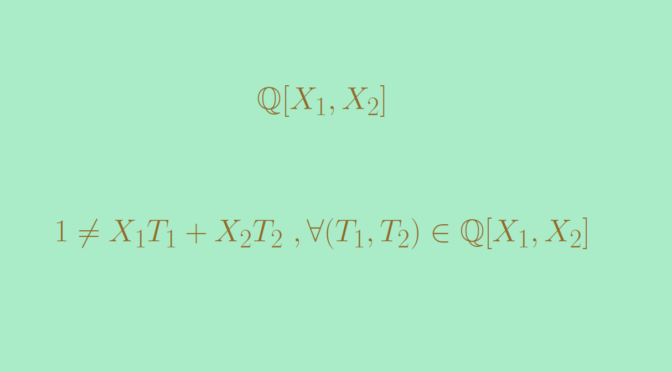# Unique factorization domain that is not a Principal ideal domain

In this article, we provide an example of a unique factorization domain – UFD that is not a principal ideal domain – PID. However, it is known that a PID is a UFD.

We take a field $$F$$, for example $$\mathbb Q$$, $$\mathbb R$$, $$\mathbb F_p$$ (where $$p$$ is a prime) or whatever more exotic.

The polynomial ring $$F[X]$$ is a UFD. This follows from the fact that $$F[X]$$ is a Euclidean domain. It is also known that for a UFD $$R$$, $$R[X]$$ is also a UFD. Therefore the polynomial ring $$F[X_1,X_2]$$ in two variables is a UFD as $$F[X_1,X_2] = F[X_1][X_2]$$. However the ideal $$I=(X_1,X_2)$$ is not principal. Let’s prove it by contradiction.

Suppose that $$(X_1,X_2) = (P)$$ with $$P \in F[X_1,X_2]$$. Then there exist two polynomials $$Q_1,Q_2 \in F[X_1,X_2]$$ such that $$X_1=PQ_1$$ and $$X_2=PQ_2$$. As a polynomial in variable $$X_2$$, the polynomial $$X_1$$ is having degree $$0$$. Therefore, the degree of $$P$$ as a polynomial in variable $$X_2$$ is also equal to $$0$$. By symmetry, we get that the degree of $$P$$ as a polynomial in variable $$X_1$$ is equal to $$0$$ too. Which implies that $$P$$ is an element of the field $$F$$ and consequently that $$(X_1,X_2) = F[X_1,X_2]$$.

But the equality $$(X_1,X_2) = F[X_1,X_2]$$ is absurd. Indeed, the degree of a polynomial $$X_1 T_1 + X_2 T_2$$ cannot be equal to $$0$$ for any $$T_1,T_2 \in F[X_1,X_2]$$. And therefore $$1 \notin F[X_1,X_2]$$.